Batch Norm and Transfer Learning — whats going on?

A commonly used technique in deep-learning is transfer-learning, whereby the learned weights of a model that was pre-trained on one dataset are used to ‘bootstrap’ training of early or all but the the final layer of a modified version of the model applied to a different dataset.

This technique allows faster training, whereby the model just learn the weights of the last fully connected layers, then applies a low learning rate finely tuned adjustment to the entire models weights.

This post explores the effect of bath-normalisation on transfer-learning based models.

Batch Norm:

Serge Ioffe and Christian Szegedy in 2015 proposed Batch Normalization (BN) where an operation is added to the model before activation which normalises the inputs (setting mean to zero and variance to one), then applies a scale and shift to preserve model performance. Two parameters, the scalar (gamma) and shift parameter (Beta) are learned by the network.

Santurkar et al. (2018) showed that rather than reducing the ‘internal covariate shift’ as proposed by it’s authors, BN actually works by making “the landscape of the corresponding optimization problem significantly more smooth. This ensures, in particular, that the gradients are more predictive and thus allows for use of larger range of learning rates and faster network convergence”. Here we explore this phenomenon.

Model

The model used here is an 18 layer ResNet by fastai, with modifications according to the Bag of Tricks for Image Classification with Convolutional Neural Networks paper by He et al. 2018

Training

PyTorch is used for model training, with code based on fastai. NB link to code will be posted here soon.

Firstly we train a model on the The Oxford-IIIT Pet Dataset, a dataset with 37 pet categories. This is trained for 40 epochs: the ‘Pre-trained model’. Next we replace the final 3 layers of the model, the AdaptiveAvgPool2d, Flatten and Linear layers and change the number of categories in the Linear layer to 10 for classifying the fastai ‘Imagewoof’ dataset.

The ‘Naive model’ is trained 5 epochs without freezing any layers without batchnorm (BN) and we see below the model performs poorly. In the image below of histograms of weights per layer, the model suffers from vanishing gradients with most gradients tending towards zero after the first layer.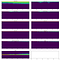Fig. 1. weights for each minibatch (x) plotted as histogram with 40 bins (y).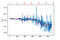Fig. 2. loss vs minibatch for training (blue) and validation (red) data for ‘Naive model’ without batch norm.

Next we trained ‘Naive model’ with batch norm for 5 epochs without freezing any layers with histograms and the loss result below: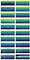Fig. 3. histograms for ‘Naive model’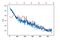Fig. 4. loss vs minibatch for training (blue) and validation (red) data for ‘Naive model’ with batch norm.

We can see the effect on batch norm on weight differences between batches in the following plot where we plot the difference between the variance for each batch. The 20 plots below correspond the the 20 layers shown in Fig. 3.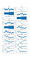Fig. 5. change in variance of weights per batch for each layer in the model. Batch Norm has a clear smoothing effect.

We then re-build the model as per above (keeping all but last 3 layers of the the ‘Pre-trained model’) freeze the weights of the network for all layers before the AdaptiveConcatPool2d layer, just train the head for 3 epochs, then we we unfroze the network and retrained the entire network for 5 epochs. We term these the ‘Freeze model’ and ‘Unfreeze model’.

We then trained a new model freezing only non batch-norm layers before the AdaptiveConcatPool2d layer, trained BN layers and head for 3 epochs, then as above we unfroze the network retrain the entire network for 5 epochs. We term this the ‘Freeze non BN model’, and ‘UnFreeze non BN model’.